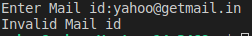# Regular Expression Methods in Python

## Regular Expression Methods in Python

In this article, I am going to discuss Regular Expression Methods in Python with examples. Please read our previous article where we discussed Regular Expressions in Python. As part of this article, we are going to discuss the following Regular Expression Important Methods in Python.

1. match()
2. fullmatch()
3. search()
4. findall()
5. finditer()
6. sub()
7. subn()
8. split()
9. compile()
##### match():

We can use the match function to check the given pattern at the beginning of the target string. If the match is available then we will get a Match object, otherwise we will get None.

Program: demo22.py

```import re
s=input("Enter pattern to check: ")
m=re.match(s, "abcabdefg")
if m != None:
print("Match is available at the beginning of the String")
print("Start Index:", m.start(), "and End Index:", m.end())
else:
print("Match is not available at the beginning of the String")```

Output1: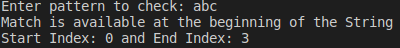Output2: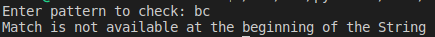##### fullmatch():

We can use fullmatch() function to match a pattern to all of target string i.e the complete string should be matched according to the given pattern. If complete string matched, then this function returns Match object otherwise it returns None.

Program: demo23.py

```import re
s=input("Enter pattern to check: ")
m=re.fullmatch(s, "ababab")
if m != None :
print("Full String Matched")
else :
print("Full String not Matched")```

Output1: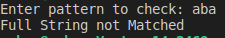Output2: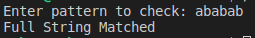##### search():

We can use the search() function to search the given pattern in the target string. If the match is available, then it returns the Match object which represents the first occurrence of the match. If the match is not available, then it returns None

Program: demo24.py

```import re
s=input("Enter pattern to check: ")
m=re.search(s, "abaaaba")
if m != None:
print("Match is available")
print("First Occurrence of match with start index:",m.start(),"and end index:", m.end())
else:
print("Match is not available")```

Output1: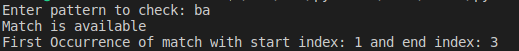Output2: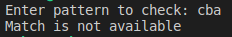##### findall():

To find all occurrences of the match. This function returns a list object which contains all occurrences.

Program: demo25.py

```import re
l=re.findall("[0-9]","a7b9c5kz")
print(l)```

Output: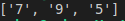##### finditer():

Returns the iterator yielding a match object for each match. On each match object we can call start(), end() and group() functions. Many examples have been covered with this method at the starting of the chapter.

Program: demo26.py

```import re
itr=re.finditer("[a-z]","a7b9c5k8z")
for m in itr:
print(m.start(),"...",m.end(),"...", m.group())```

Output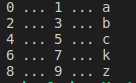##### sub():

sub means substitution or replacement

re.sub(regex, replacement, targetstring)

In the target string every matched pattern will be replaced with provided replacement.

Program: demo27.py

```import re
s=re.sub("[a-z]","#","a7b9c5k8z")
print(s)```

Output: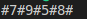##### subn():

It is exactly the same as sub except it can also return the number of replacements. This function returns a tuple where the first element is the result string and second element is the number of replacements.

(resultstring, number of replacements)

Program: demo28.py

```import re
t=re.subn("[a-z]","#","a7b9c5k8z")
print(t)
print("The Result String:", t)
print("The number of replacements:", t)```

Output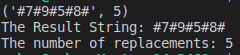##### split():

If we want to split the given target string according to a particular pattern then we should go for the split() function. This function returns a list of all tokens.

Program: demo29.py

```import re
l=re.split("," , "KGF,BB1,BB2")
print(l)
for t in l:
print(t)```

Output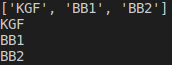Note: ^ symbol: We can use ^ symbol to check whether the given target string starts with our provided pattern or not. If the target string starts with Learn then it will return Match object, otherwise returns None.

res=re.search(“^Learn”, s)

Program: demo31.py

```import re
s="Learning Python is Very Easy"
res=re.search("^Learn", s)
if res !=None:
print("Target String starts with Learn")
else:
print("Target String Not starts with Learn")```

Output: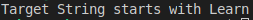##### \$ symbol:

We can use \$ symbol to check whether the given target string ends with our provided pattern or not. If the target string ends with Easy then it will return Match object, otherwise returns None.

res=re.search(“Easy\$”, s)

Program: demo32.py

```import re
s="Learning Python is Very Easy"
res=re.search("Easy\$", s)
if res !=None:
print("Target String ends with Easy")
else:
print("Target String Not ends with Easy")```

Output: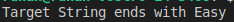If we want to ignore case then we have to pass 3rd argument re.IGNORECASE for search() function.

res = re.search(“easy\$”, s, re.IGNORECASE)

###### Program: demo33.py
```import re
s="Learning Python is Very Easy"
res=re.search("easy\$", s, re.IGNORECASE)
if res !=None:
print("Target String ends with Easy by ignoring case")
else:
print("Target String Not ends with Easy by ignoring case")```

Output: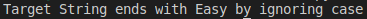#### SAMPLE PROGRAMS

##### PROGRAM 1:

Let’s consider the following requirement to create a regex object.

1. The allowed characters are a-z, A-Z, 0-9,#
2. The first character should be a lower-case alphabet symbol from a to k
3. The second character should be a digit divisible by 3.
4. The length of the identifier should be at least 2.

Regular expression: [a-k][a-zA-Z0-9#]*.

Write a python program to check whether the given string is following requirement1 rules or not?

```import re
s=input("Enter string:")
m=re.fullmatch("[a-k][a-zA-Z0-9#]*",s)
if m!= None:
print(s, "Entered regular expression is matched")
else:
print(s, " Entered regular expression is not matched ")```

Output1: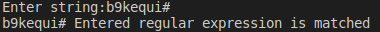Output2: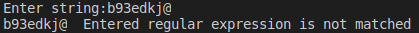Output: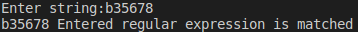##### PROGRAM 2:

Write a Regular Expression to represent all 10 digit mobile numbers

Rules:

1. Every number should contain exactly 10 digits
2. The first digit should be 7 or 8 or 9

Regular Expressions:
[7-9][0-9][0-9][0-9][0-9][0-9][0-9][0-9][0-9][0-9]
or
[7-9][0-9]{9}
or
[7-9]\d{9}

```import re
n=input("Enter number:")
m=re.fullmatch("[7-9]\d{9}",n)
if m!=None:
print("Valid Mobile Number")
else:

Output1: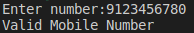Output2: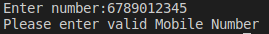##### PROGRAM 3:

Write a python program to extract all mobile numbers present in input.txt where numbers are mixed with normal text data

```import re
f1=open("input.txt", "r")
f2=open("output.txt", "w")
for line in f1:
items = re.findall("[7-9]\d{9}",line)
for n in items:
f2.write(n+"\n")
print("Extracted all Mobile Numbers into output.txt")
f1.close()
f2.close()```

Output: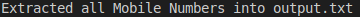input.txt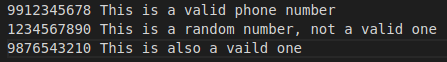output.txt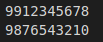##### PROGRAM 4:

Write a Python Program to check whether the given mail id is valid gmail id or not?

```import re
s=input("Enter Mail id:")
m=re.fullmatch("\w[a-zA-Z0-9_.]*@gmail[.]com", s)
if m!=None:
print("Valid Mail Id")
else:
print("Invalid Mail id")```

Output1: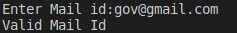Output2: